Learn US

# How to Find the Areas of Various Shapes in Geometry?

741 views

 1 Introduction 2 What is “Area”? 3 Grid Technique 4 Formulas to calculate Areas of different shapes 5 Real-Life Examples 6 What we learned 7 Summary

14 December 2020

## Introduction

Our World is full of amazing shapes.

Circles & Ovals
Square & Rectangles,
Stars & Triangles.

It's fun to know about different shapes and their structure.
You use a lot of objects with different kinds of shapes in your daily and work life.

In this article, you will find out different types of shapes and how to calculate the area of shapes.

Geometry is a science, in which you study types of shapes and their dimensions. It has developed formulas to find out the area of specific shapes.

Many times, the need arises to calculate the surface area covered by these objects. Let's start with knowing how the area is defined.

### How to Find the Areas of Various Shapes in Geometry-PDF

Learn about the different shapes and how to calculate their areas, Grid Technique and Real-Life Examples. Here is a downloadable PDF to explore more.

 📥 How to Find the Areas of Various Shapes in Geometry-PDF Download

## What is “Area”?

Word "Area" means vacant surface in Latin. The area can be defined as the amount of space covered by a flat surface of a particular shape.

It is measured in terms of the "number of" square units.  (Square Centimeters, Square Inches, Square feet, etc).

Knowing the area of shape of the surface helps us in daily life. It helps to communicate or give information in practical life.

For example, the area of a plot can be used to calculate the amount of surface available for construction or the area of the room that can be calculated to decide the size of the carpet to be used.

Most objects or shapes have edges and corners. The length and width of these edges are considered to calculate the area of a specific shape.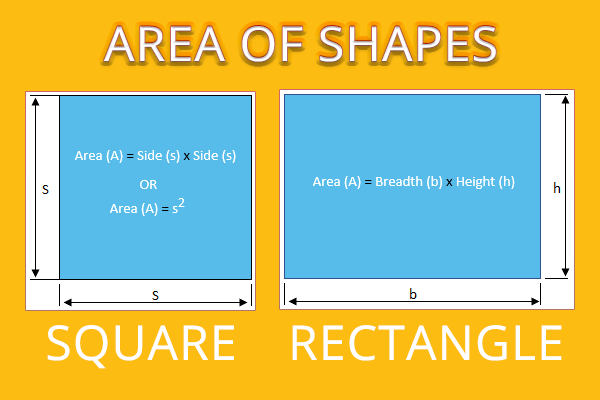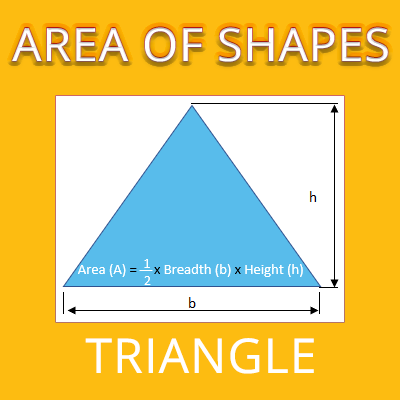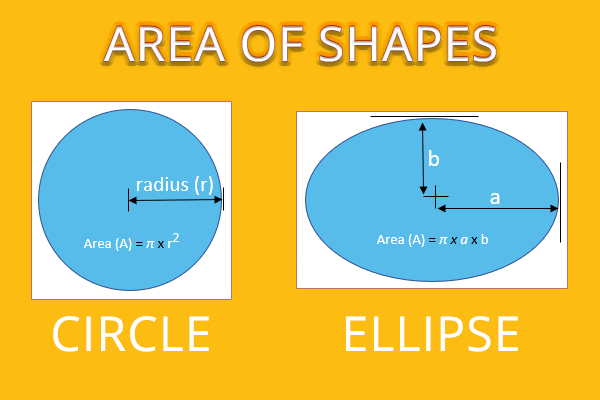## How to use the Grid Technique to find Area?

Grid techniques can help us to have a better understanding of these formulas.

Let us draw a shape on a scaled grid. One can find the area by counting the number of squares covering the inside area of the shape.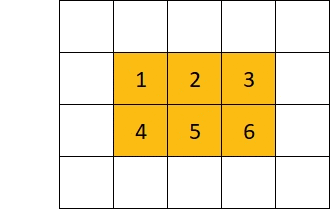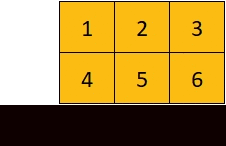In this picture, there are 6 grid squares in a rectangle. One should know the dimensions of the grid square.

It could be in centimeters, inches, or feet. Suppose the width of 1 square is 1cms, then the area of the rectangle as per this picture would be 6 Square cms. The idea is, how many squares cover the inside space of shape.

## Formulas to calculate Areas of different shapes

### Square

For calculating the area for a square, one just needs to know the length of one side of the square and multiply this number by itself.

Eg. if the length of the square is 4 cm, then the area is 4 cms x 4cm = 16 sq cms.### Rectangle

To find the area of a rectangle, one needs to know the length and width of it.

Simply by multiplying its length with width, the area can be calculated

Eg. The Length of the rectangle is 4 cm and the width is 5 cm.

So area would be : 4cms x 5 cms = 20 sq cms.

### Triangle

Look at the picture given below. If you observe, the triangle is half of a square or parallelogram. Naturally, its area would be half of the square/parallelogram.

i.e. ( Length x height ) /2
Now, the height of the triangle can be measured by drawing a straight line (right-angled line) from the base of the triangle to the apex (top corner of the triangle).Here in the above example, the area of the triangle would be 4 × 3 /2 = 6 sq cms.

### Circle

To get the area of the circle, one should know its diameter or radius.

The radius of the circle is the length of the line from the center point of the circle towards its edge. Radius is half of the diameter.

Diameter is the line drawn between two edges of circles that are passing through the center of the same circle.

The formula to find the area of the circle is π × r2  where the value of π(3.142) is constant.

Hence the area of a circle is: 3.142 × Radius of circle2Eg. The radius of the circle is 3 cms. then the area would be: 3.142 × 32 = 28.278 sq cms.

## Some Real-Life Examples on Area of Shapes

You can apply these formulas to get the area of different shapes.

1. Suppose, Jini got 4 pieces of one medium size of the pizza and Jony got 3 pieces of another large size pizza. How do Jini and Jony will know who got more pizza?First step: we need to calculate the area of both Pizza. For that, we will consider the Radius of its diameter as Pizza has a circle shape.

Suppose Medium size Pizza has a radius of 10 cms. Its area would be
3.142 × 102 as per formula.
i.e. π × R2
The area would be :
314.2 sq. cms.

Similarly, a large size pizza has a radius of 16 cms, the area would be:
3.142 × 162 =
804.352 sq. cms.

Now, when we have got the area, let's see how many shares of pizza Gini and Jon has got.

Gini has got 4 equal pieces out of 6 of medium size pizza, which has an area of  62.84 sq. cms.
So Gini’s share is 314.2 / 6 × 4 = 209.466 sq. cms.

And Jony has got 3 equal size pieces out of 6 of medium size pizza. Which has an area of 100.544 sq. cms
His share would be 804.352 / 6 × 3 = 402.176 sq. cms.

From the above, we can say Johnny has got more pizza.

 Johnny has got more pizza

2. One needs to find out the area of a wall to carry painting work.The first step is to calculate the entire wall area by measuring height and width.

Subtracting Window / Door area by measuring the height and width of the Window/ Door.

And one can find out the exact area of work.

Suppose Height is 11Sq Feet and width is 10 Sq Feet.

The area of the wall would be: 11 feet × 10 feet (Height × Width) = 110sq feet

From this area, you need to exclude the area of the Window.

Let's calculate the area of the window, by considering the height and width of the Window.

The same would be 3 feet in height and 4 feet in width.
So the area of the window would be 3 feet × 4feet = 12 feet.

Hence, the total work area would be:

 110 sq feet - 12 sq feet = 98 sq feet.

Shown above is an example of how to calculate shapes of the area which are useful in day to day life.

There are various other areas where we need to calculate areas of shapes. Formulas are helpful to get the area of different and complex shapes.

## What we learned

The below-given table below contains some basic shapes and formulae to calculate their areas.

 Shape Drawing Formula Squarea²  ( “a” stand for the length of one side of the square) RectangleLength × Width Triangle½ (Base × height) Circleπ x R2 ( “π” Stand for - 3.142 & “R” stand for - Radius of circle)

## Summary

The area of shapes is a very important topic for exams as well as in practical life, it has many applications.

From the clay tablets of the Babylonian Era, showing the area calculation of the trapezoid to the modern-day formulae for the various shapes, calculating area has not only been a fascinating area of study but also has infinite practical applications.

Same shapes having different areas and different shapes having the same areas, all need to be calculated for various purposes like construction of buildings, painting areas, putting tiles, selling items and the list is endless.

For simple shapes, you can use the Grid Technique to find the area otherwise you can simply use the formulas.

If you think your friends can benefit from this article, feel free to share it with them!

Cuemath, student-friendly mathematics and coding platform, conducts regular Online Live Classes for academics and skill-development, and their Mental Math App, on both iOS and Android, is a one-stop solution for kids to develop multiple skills.

Check out the fee structure for all grades and book a trial class today!

## What is Area?

The area can be defined as the amount of space covered by a flat surface of a particular shape.

## What is the Grid Technique?

Grid techniques can help us to have a better understanding of these formulas for finding area. Draw a shape on a scaled grid. One can find the area by counting the number of squares covering the inside area of the shape

## What are the different types of Shapes?

Various types of shapes are:

• Triangle
• Square
• Rectangle
• Pentagon
• Hexagon etc.

## What is the Area of Triangle?

If you observe, the triangle is half of a square or parallelogram. Naturally, its area would be half of the square/parallelogram i.e. ( Length x height ) /2

## What is the Area of Square?

For calculating the area for a square, one just needs to know the length of one side of the square and multiply this number by itself.

## What is the Area of Rectangle?

To find the area of a rectangle, one needs to know the length and width of it. Simply by multiplying its length with width, the area can be calculated

## What is the Area of Circle?

The formula to find the area of the circle is π × r2  where the value of π(3.142) is constant. Here r is the radius of the circle.

## External References

GIVE YOUR CHILD THE CUEMATH EDGE
Access Personalised Math learning through interactive worksheets, gamified concepts and grade-wise courses